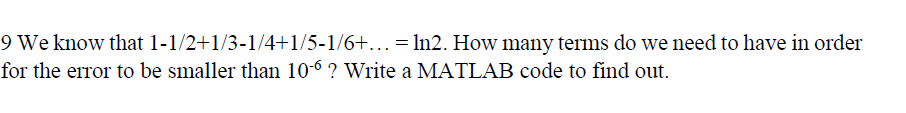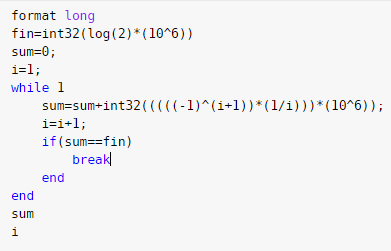# Question & Answer: We know that 1 – 1/2 + 1/3 – 1/4 + 1/5 – 1/6 + = ln2. How many terms do we need to have in order for the error to be smaller than 10^-6 ?…..We know that 1 – 1/2 + 1/3 – 1/4 + 1/5 – 1/6 + = ln2. How many terms do we need to have in order for the error to be smaller than 10^-6 ? Write a MATLAB code to find out.

SOURCE CODE:
format long
fin=int32(log(2)*(10^6))
sum=0;
i=1;
while 1
sum=sum+int32(((((-1)^(i+1))*(1/i)))*(10^6));
i=i+1;
if(sum==fin)
break
end
end
sum
i

SCREENSHOTS: